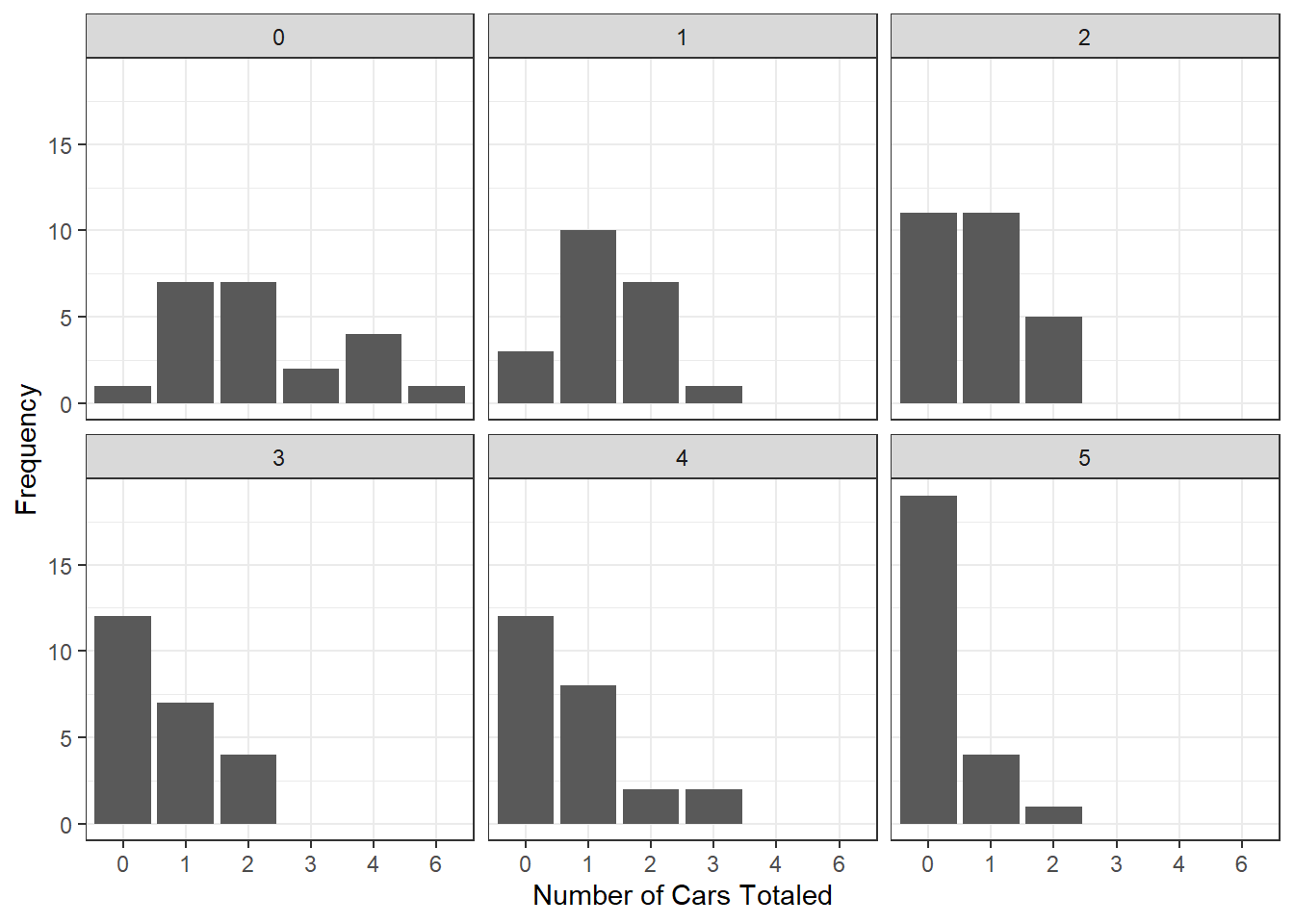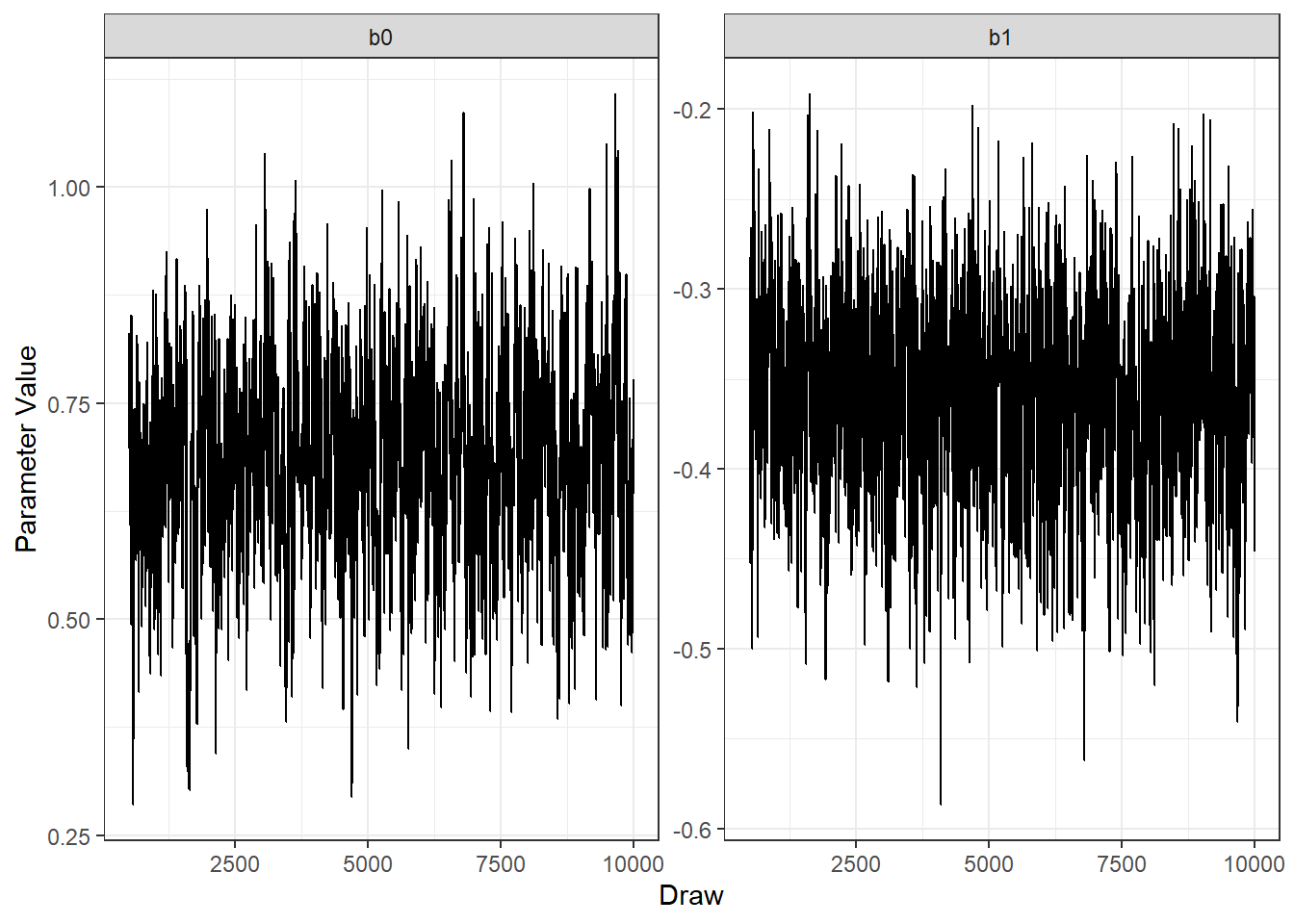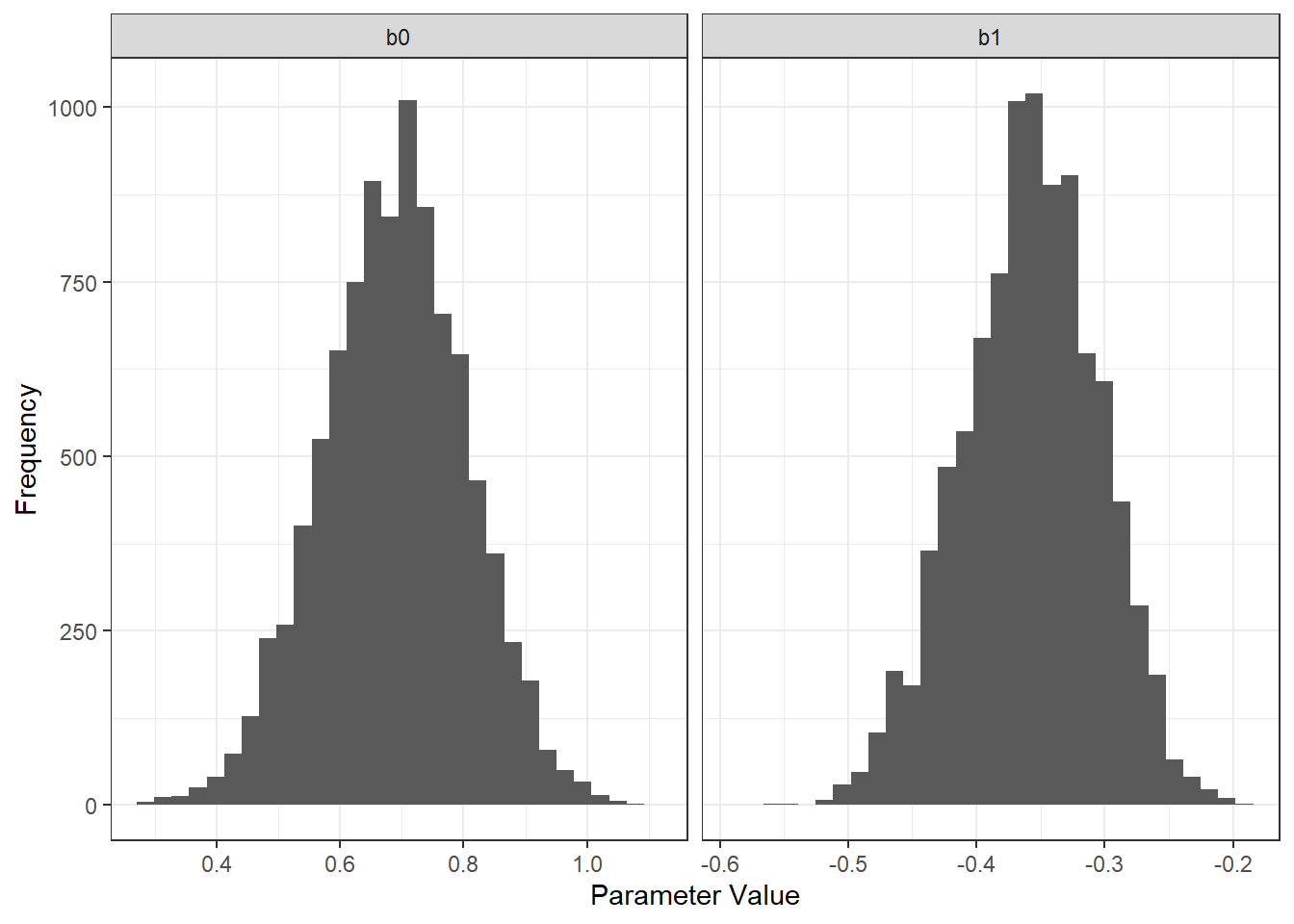# Data first

Let’s begin by describing the data and our generative model.

We have yearly data that follows a Poisson distribution. Specifically, the data describes the number of totaled cars resulting from collisions that occurred in a heavily-trafficked highway.

$ncars_{year} \sim Poisson(\lambda_{year})$ The rate parameter $$\lambda_{year}$$ must be positive, so we’ll express it as the combination of two real numbers, as follows:

$\lambda_{year} = exp(\beta_0 + x_{year} * \beta_1)$ $\beta_0 \sim Normal(\mu,\sigma)$ $\beta_1 \sim Normal(\mu,\sigma)$ Let’s import some helper packages and generate the data

require(tidyverse)
theme_set(theme_bw())

#First, set the ground truth
set.seed(1) #For reproducibility
b0 <- 0.6
b1 <- -0.3
years <- 0:5
nf <- function(x) runif(1, min = 20, max = 30) #to randomize the number of collisions per year

cardat <- tibble(year = years, l = exp(b0 + year*b1)) %>% rowwise() %>%
mutate(ncars = list(rpois(nf(), lambda = l))) %>%
unnest(ncars)

#Let's look at the data
carplot <- cardat %>% ggplot(aes(x = as.factor(ncars))) +
geom_bar() + facet_wrap(~year) +
labs(x = 'Number of Cars Totaled', y = 'Frequency')
carplot# The MCMC algorithm

The MCMC algorithm is quite clever. It allows us to get samples from the posterior distribution in a systematic way, while also allowing for a great deal of exploration of the parameter space. The algorithm goes like this:

1 - Propose an initial proposal $$\theta$$. For N-1 samples:

2 - Calculate the posterior likelihood of that proposal ($$P(y|\theta)P(\theta)$$)

3 - Make a new proposal ($$\theta'$$), based on the previous proposal with some noise ($$\theta' = Normal(\theta, \sigma)$$)

4 - Calculate the posterior likelihood of the new proposal ($$P(y|\theta')P(\theta')$$)

5 - Accept the proposal if the ratio between the posterior likelihoods is larger than a criterion.

6 - Go to 3 until done.

To tackle this algorithm, we will first implement the posterior likelihood function, as it will greatly simplify our algorithm code.

Recall that our model is composed by two parameters, $$\beta_0$$ and $$\beta_1$$, both of which are normally distributed. We can use $$\mu = 0$$ and $$\sigma = 1$$ as parameters for their normal prior (although more informative priors would be better). In practice, we will get the likelihood of these parameters using dnorm.

Also recall that our generative model is a Poisson model. We will compute the likelihood of the data, given the model, using dpois.

Let’s write the function now. You might want to brush up on your logarithm fundamentals, as we will use log-likelihoods instead of raw likelihoods to avoid numerical issues. In case you are rusty, remember that $$ab = log(a)+log(b)$$ and that $$a/b = log(a)-log(b)$$.

posterior_loglik <- function(pars, ncars, years, pmu = 0, psigma = 1){
#Calculate lambdas
lambdas = exp(pars + pars*years)
ll_data_model = dpois(ncars, lambdas, log = T) #note the log
#Now the priors
ll_prior = dnorm(pars, mean = pmu, sd = psigma, log = T)
#Now let's sum everything together
return(sum(ll_data_model, ll_prior))
}

And now, let’s implement the MCMC algorithm. We will run 10000 samples. This might be a little bit tricky to understand, depending on your programming proficiency.

nsamples <- 10000 #number of samples
proposal_noise <- .1 #How much noise we'll add to the old proposal in order to create a new one
sampledat <- array(NA, dim = c(nsamples, 2)) #Array that will contain our samples.
old_proposal <- rnorm(2) #First proposal, name does not make much sense, but will in a few lines
#Let's calculate the posterior loglikelihood of this proposal
old_posterior <- posterior_loglik(pars = old_proposal,
ncars = cardat$ncars, years = cardat$year)
sampledat[1, ] <- old_proposal #Saving the first proposal

for (samp in 1:nsamples){
#Generate a proposal
new_proposal <- rnorm(2, mean = old_proposal, sd = proposal_noise)
#Calculate its posterior loglikelihood
new_posterior <- posterior_loglik(pars = new_proposal,
ncars = cardat$ncars, years = cardat$year)
llratio <- new_posterior-old_posterior
if (llratio > log(runif(1))){
#We accept the new proposal
sampledat[samp, ] = new_proposal #save the proposal
#It becomes the old proposal for the next loop
old_proposal = new_proposal
old_posterior = new_posterior
}else{
#We reject the new proposal
sampledat[samp, ] = old_proposal #save the proposal
}
}

We can now visualize our posterior distributions. First, let’s look at how well we explored the space.

colnames(sampledat) <- c('b0', 'b1')
sampledat <- sampledat %>% as.data.frame() %>% mutate(draw = 1:n()) %>%
pivot_longer(cols = c('b0', 'b1'), names_to = 'parameter', values_to = 'value')
draws <- sampledat %>%
filter(draw > 500) %>% #consider it a burnin period
ggplot(aes(x = draw, y = value)) +
geom_line() + facet_wrap(~parameter, scales = 'free_y') +
labs(x = 'Draw', y = 'Parameter Value')
drawsThat’s pretty good. We want to move around in parameter space so we can get a good idea of what our posterior looks like. Now, let’s look at the posterior distributions themselves.

dists <- sampledat %>%
filter(draw > 500) %>% #consider it a burnin period
ggplot(aes(x = value)) +
geom_histogram() + facet_wrap(~parameter, scales = 'free_x') +
labs(x = 'Parameter Value', y = 'Frequency')
distsCongratulations! You know understand how MCMC works! Pat yourself in the back for now. Done? Now go watch a lecture by Michael Betancourt on why nobody uses MCMC anymore.